Publicité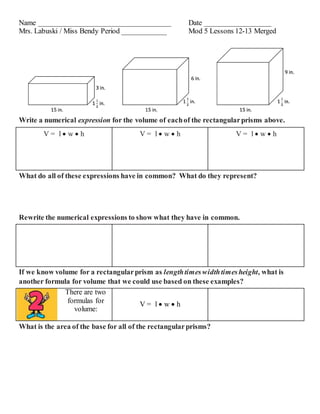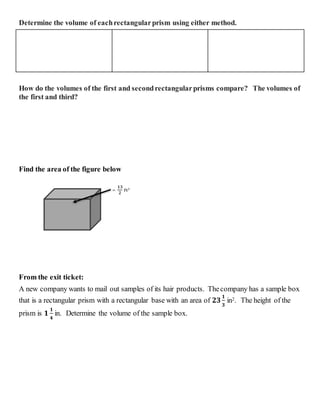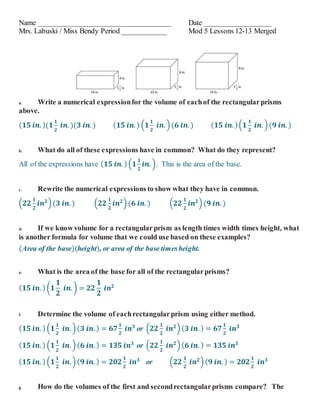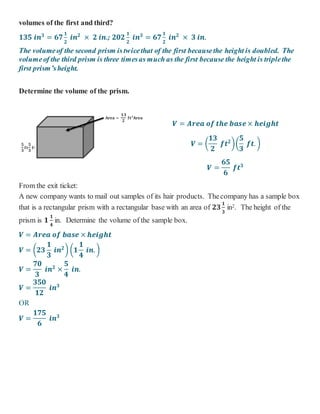Prochain SlideShare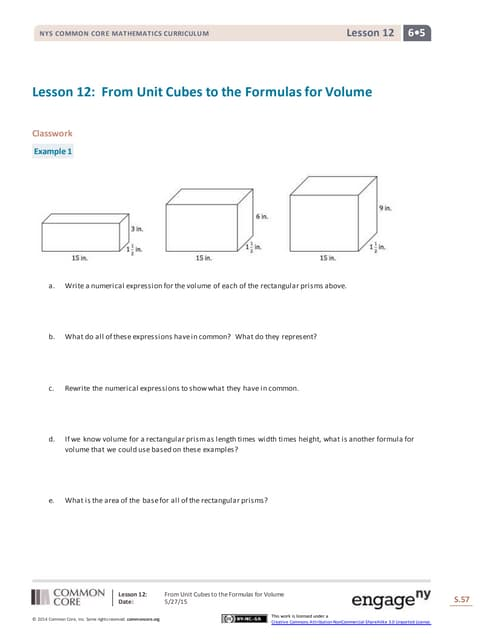G6 m5-c-lesson 12-s
Chargement dans ... 3
1 sur 4
Publicité

### Lessons 12 13 merged

1. Name ____________________________________ Date __________________ Mrs. Labuski / Miss Bendy Period ____________ Mod 5 Lessons 12-13 Merged Write a numerical expression for the volume of eachof the rectangularprisms above. V = l  w  h V = l  w  h V = l  w  h What do all of these expressions have in common? What do they represent? Rewrite the numerical expressions to show what they have in common. If we know volume for a rectangularprism as lengthtimeswidthtimesheight, what is another formula for volume that we could use based on these examples? There are two formulas for volume: V = l  w  h What is the area of the base for all of the rectangularprisms?
2. = 𝟏𝟑 𝟐 𝐟𝐭 𝟐 Determine the volume of eachrectangularprism using either method. How do the volumes of the first and secondrectangularprisms compare? The volumes of the first and third? Find the area of the figure below From the exit ticket: A new company wants to mail out samples of its hair products. Thecompany has a sample box that is a rectangular prism with a rectangular base with an area of 𝟐𝟑 𝟏 𝟑 in2. The height of the prism is 𝟏 𝟏 𝟒 in. Determine the volume of the sample box.
3. Name ____________________________________ Date __________________ Mrs. Labuski / Miss Bendy Period ____________ Mod 5 Lessons 12-13 Merged a. Write a numerical expressionfor the volume of eachof the rectangularprisms above. ( 𝟏𝟓 𝒊𝒏. )(𝟏 𝟏 𝟐 𝒊𝒏. )(𝟑 𝒊𝒏. ) ( 𝟏𝟓 𝒊𝒏. ) (𝟏 𝟏 𝟐 𝒊𝒏. )(𝟔 𝒊𝒏. ) ( 𝟏𝟓 𝒊𝒏. )(𝟏 𝟏 𝟐 𝒊𝒏. )(𝟗 𝒊𝒏. ) b. What do all of these expressions have in common? What do they represent? All of the expressions have ( 𝟏𝟓 𝒊𝒏. ) (𝟏 𝟏 𝟐 𝒊𝒏. ). This is the area of the base. c. Rewrite the numerical expressions to show what they have in common. (𝟐𝟐 𝟏 𝟐 𝒊𝒏 𝟐 )(𝟑 𝒊𝒏. ) (𝟐𝟐 𝟏 𝟐 𝒊𝒏 𝟐 )(𝟔 𝒊𝒏. ) (𝟐𝟐 𝟏 𝟐 𝒊𝒏 𝟐 )(𝟗 𝒊𝒏. ) d. If we know volume for a rectangularprism as length times width times height, what is another formula for volume that we could use based on these examples? (Area of the base)(height), or area of the base timesheight. e. What is the area of the base for all of the rectangularprisms? ( 𝟏𝟓 𝒊𝒏. ) (𝟏 𝟏 𝟐 𝒊𝒏. ) = 𝟐𝟐 𝟏 𝟐 𝒊𝒏 𝟐 f. Determine the volume of eachrectangularprism using either method. ( 𝟏𝟓 𝒊𝒏. ) (𝟏 𝟏 𝟐 𝒊𝒏. )( 𝟑 𝒊𝒏. ) = 𝟔𝟕 𝟏 𝟐 𝒊𝒏 𝟑 or (𝟐𝟐 𝟏 𝟐 𝒊𝒏 𝟐 )( 𝟑 𝒊𝒏. ) = 𝟔𝟕 𝟏 𝟐 𝒊𝒏 𝟑 ( 𝟏𝟓 𝒊𝒏. ) (𝟏 𝟏 𝟐 𝒊𝒏. )( 𝟔 𝒊𝒏. ) = 𝟏𝟑𝟓 𝒊𝒏 𝟑 or (𝟐𝟐 𝟏 𝟐 𝒊𝒏 𝟐 )( 𝟔 𝒊𝒏. ) = 𝟏𝟑𝟓 𝒊𝒏 𝟑 ( 𝟏𝟓 𝒊𝒏. ) (𝟏 𝟏 𝟐 𝒊𝒏. )( 𝟗 𝒊𝒏. ) = 𝟐𝟎𝟐 𝟏 𝟐 𝒊𝒏 𝟑 or (𝟐𝟐 𝟏 𝟐 𝒊𝒏 𝟐 )( 𝟗 𝒊𝒏. ) = 𝟐𝟎𝟐 𝟏 𝟐 𝒊𝒏 𝟑 g. How do the volumes of the first and secondrectangularprisms compare? The
4. 𝐀𝐫𝐞𝐚 = 𝟏𝟑 𝟐 𝐟𝐭 𝟐 𝐀𝐫𝐞𝐚 𝟓 𝟑 𝐟𝐭 𝟓 𝟑 𝐟𝐭 volumes of the first and third? 𝟏𝟑𝟓 𝒊𝒏 𝟑 = 𝟔𝟕 𝟏 𝟐 𝒊𝒏 𝟐 × 𝟐 𝒊𝒏.; 𝟐𝟎𝟐 𝟏 𝟐 𝒊𝒏 𝟑 = 𝟔𝟕 𝟏 𝟐 𝒊𝒏 𝟐 × 𝟑 𝒊𝒏. The volumeof the second prism istwicethat of the first becausethe height is doubled. The volumeof the third prism is three timesas much as the first because the height is triplethe first prism’sheight. Determine the volume of the prism. 𝑽 = 𝑨𝒓𝒆𝒂 𝒐𝒇 𝒕𝒉𝒆 𝒃𝒂𝒔𝒆× 𝒉𝒆𝒊𝒈𝒉𝒕 𝑽 = ( 𝟏𝟑 𝟐 𝒇𝒕 𝟐 )( 𝟓 𝟑 𝒇𝒕. ) 𝑽 = 𝟔𝟓 𝟔 𝒇𝒕 𝟑 From the exit ticket: A new company wants to mail out samples of its hair products. Thecompany has a sample box that is a rectangular prism with a rectangular base with an area of 𝟐𝟑 𝟏 𝟑 in2. The height of the prism is 𝟏 𝟏 𝟒 in. Determine the volume of the sample box. 𝑽 = 𝑨𝒓𝒆𝒂 𝒐𝒇 𝒃𝒂𝒔𝒆 × 𝒉𝒆𝒊𝒈𝒉𝒕 𝑽 = (𝟐𝟑 𝟏 𝟑 𝒊𝒏 𝟐 ) (𝟏 𝟏 𝟒 𝒊𝒏. ) 𝑽 = 𝟕𝟎 𝟑 𝒊𝒏 𝟐 × 𝟓 𝟒 𝒊𝒏. 𝑽 = 𝟑𝟓𝟎 𝟏𝟐 𝒊𝒏 𝟑 OR 𝑽 = 𝟏𝟕𝟓 𝟔 𝒊𝒏 𝟑
Publicité